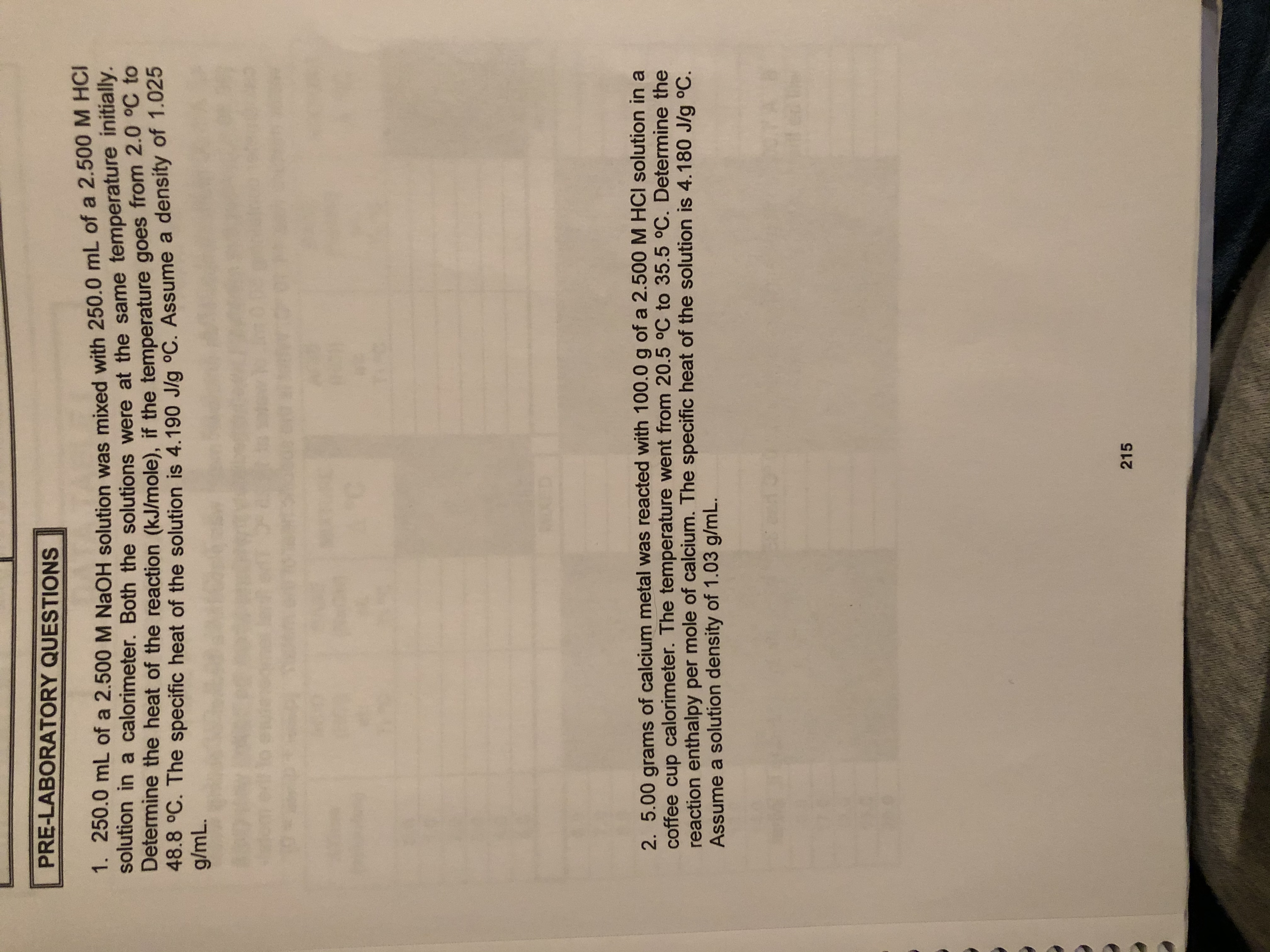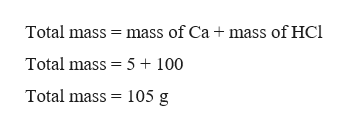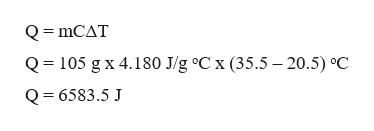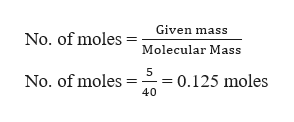# PRE-LABORATORY QUESTIONS1. 250.0 mL of a 2.500 M NaOH solution was mixed with 250.0 mL of a 2.500 M HCIltion in a calorimeter. Both the solutions were at the same temperature initially.Determine the heat of the reaction (kJ/mole), if the temperature goes from 2.0 °C to48.8 °C. The specific heat of the solution is 4.190 Jlg °C. Assume a density of 1.025g/mL.KETD2. 5.00 grams of calcium metal was reacted with 100.0 g of a 2.500 M HCI solution in acoffee cup calorimeter. The temperature went from 20.5 °C to 35.5 °C. Determine thereaction enthalpy per mole of calcium. The specific heat of the solution is 4.180 J/g oC.Assume a solution density of 1.03 g/mL.215

Question
3 views

Question 2help_outlineImage TranscriptionclosePRE-LABORATORY QUESTIONS 1. 250.0 mL of a 2.500 M NaOH solution was mixed with 250.0 mL of a 2.500 M HCI ltion in a calorimeter. Both the solutions were at the same temperature initially. Determine the heat of the reaction (kJ/mole), if the temperature goes from 2.0 °C to 48.8 °C. The specific heat of the solution is 4.190 Jlg °C. Assume a density of 1.025 g/mL. KET D 2. 5.00 grams of calcium metal was reacted with 100.0 g of a 2.500 M HCI solution in a coffee cup calorimeter. The temperature went from 20.5 °C to 35.5 °C. Determine the reaction enthalpy per mole of calcium. The specific heat of the solution is 4.180 J/g oC. Assume a solution density of 1.03 g/mL. 215 fullscreen
check_circle

Step 1

The total mass of solution is calculated as,help_outlineImage TranscriptioncloseTotal mass mass of Ca mass of HCl Total mass 5 100 Total mass 105 g fullscreen
Step 2

The total heat can be calculated as,help_outlineImage TranscriptioncloseQ mCAT 105 g x 4.180 J/g °C x (35.5 -20.5) °C Q Q 6583.5 J fullscreen
Step 3

It is required to calculate the enthalpy per mole of calcium, hence calc...help_outlineImage TranscriptioncloseGiven mass No. of moles Molecular Mass 5 0.125 moles No. of moles 40 fullscreen

### Want to see the full answer?

See Solution

#### Want to see this answer and more?

Solutions are written by subject experts who are available 24/7. Questions are typically answered within 1 hour.*

See Solution
*Response times may vary by subject and question.
Tagged in

### Chemical Thermodynamics